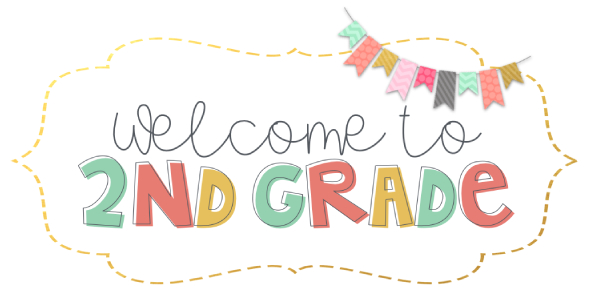# Rocketry & Space Travel Chapter 3 Science Quiz For 2nd Grade

31 Questions | Total Attempts: 202SettingsCreate your own Quiz2nd Quarter Test for Science 7. Chapter 3 - Rocketry & Space Travel

• 1.
True or False.  The aperture is the opening of the telescope which lets in light.
• A.

True

• B.

False

• 2.
Newton's First Law: an object at rest tends to ______________________________.
• A.

React more rapidly to gravitational pull than an object in motion

• B.

Have an opposite and equal reaction

• C.

Drift slowly at first, then more quickly when an outside force is applied

• D.

Stay at rest until acted upon by an outside force

• 3.
F = m a means
• A.

Force = mass x appreciation

• B.

Force = mass x acceleration

• C.

Flux = mass x acceleration

• D.

Flux = mass x appreciation

• 4.
F = m ais used to calculate the ___________ of an object.
• A.

Flux

• B.

Fracture

• C.

Force

• D.

Frequency

• E.

First Law of Newton

• 5.
A/An __________ orbit is _________ and the satellite does not remain the same distance away from the planet.
• A.

Elliptical / not even

• B.

Elliptical / even

• C.

Circular / not even

• D.

Circular / even

• 6.
A/An __________ orbit is _________ and the satellite remains the same distance away from the planet.
• A.

Elliptical / not even

• B.

Elliptical / even

• C.

Circular / not even

• D.

Circular / even

• 7.
In a/an _________________ orbit the closest point of the orbit is the ___________ and the point furthest is called the ______________.
• A.

Circular / perigee / apogee

• B.

Elliptical / perigee / apogee

• C.

Circular / apogee / perigee

• D.

Elliptical / apogee / perigee

• 8.
The most famous space telescope is called the _________________ Space Telescope
• A.

Hemble

• B.

Himble

• C.

Humble

• D.

Hamble

• E.

None of the above

• 9.
The __________ landers, which were sent to __________ , used __________ to collect __________ rock and soil.
• A.

Lunar / Moon / robotic arms / Lunar

• B.

Combustion / Moon / highly trained astronauts / Lunar

• C.

Viking / Mars / robotic arms / Martian

• D.

Voyager / Mars / highly trained astronauts / Martian

• 10.
All fires need these three things.
• A.

Fuel, heat and exhaust

• B.

Oxygen, heat and gravity

• C.

Exhaust, oxygen and fuel

• D.

Fuel, heat and oxygen

• E.

Oxygen, fuel and gravity

• 11.
A/An ___________________ telescope uses a large mirror.
• A.

Reflecting

• B.

Refracting

• C.

Magnifying

• D.

Aperature

• 12.
A/An ___________________ telescope uses only lenses to produce images.
• A.

Reflecting

• B.

Refracting

• C.

Magnifying

• D.

Aperature

• 13.
The Hubble Space Telescope is an example of a _________________ telescope.
• A.

Reflecting

• B.

Refracting

• C.

Magnifying

• D.

Aperature

• 14.
_____________________ are unmanned space vehicles sent out to gather information about planets.
• A.

Refracting lenses

• B.

Satellite perigees

• C.

Robotic landers

• D.

Space probes

• 15.
In order for a rocket to get as high off Earth as it can it must fight ___________.
• A.

Oxygen

• B.

Gravity

• C.

Atmospheric pressure

• D.

Thrust

• 16.
The ________________________ is the only equipment lost on each mission of the U.S. Space Shuttle.
• A.

Solid rocket booster

• B.

Cargo

• C.

External fuel tank

• D.

Internal fuel tank

• E.

None of the above

• 17.
The U.S. Space Shuttle is a significant development in space exploration because it is ______________.
• A.

Inexpensive

• B.

Extremely expensive

• C.

Reusable

• D.

Not reusable

• 18.
The Voyager 2 space probe visited which four planets.
• A.

Mercury, Venus, Mars and Jupiter

• B.

Mars, Jupiter, Neptune and Pluto

• C.

Jupiter, Saturn, Uranus and Neptune

• D.

Uranus, Mercury, Venus and Saturn

• 19.
True or False. The Galileo probe gathered much information about Jupiter and its moons before returning to earth.
• A.

True

• B.

False

• 20.
True or False.  Some space probes have left our Solar System.
• A.

True

• B.

False

• 21.
The first person in space was ____________________.
• A.

Neil Armstrong, an American astronaut

• B.

Yuri Gagarin, a Soviet cosmonaut

• C.

Buzz Aldrin, an American astronaut

• D.

Johannes Kepler, a German astronaut

• 22.
The first person to walk on the moon was ____________________.
• A.

Neil Armstrong, an American astronaut

• B.

Yuri Gagarin, a Soviet cosmonaut

• C.

Buzz Aldrin, an American astronaut

• D.

Johannes Kepler, a German astronaut

• 23.
The force of an object is equal to its mass times its acceleration is ____________________.
• A.

Newton's First Law

• B.

Newton's Second Law

• C.

Newton's Third Law

• D.

Newton's Fourth Law

• E.

Newton's Fifth Law

• 24.
__________________ confirmed the findings of Copernicus, that the Earth orbits the sun.
• A.

Gagarin

• B.

Newton

• C.

Kepler

• D.

Galileo

• 25.
True or False.  The U.S. Space Shuttle has a remote-controlled robotic arm that is used to launch, retrieve, and repair satellites in space.
• A.

True

• B.

False

Related TopicsBack to top
×

Wait!
Here's an interesting quiz for you.